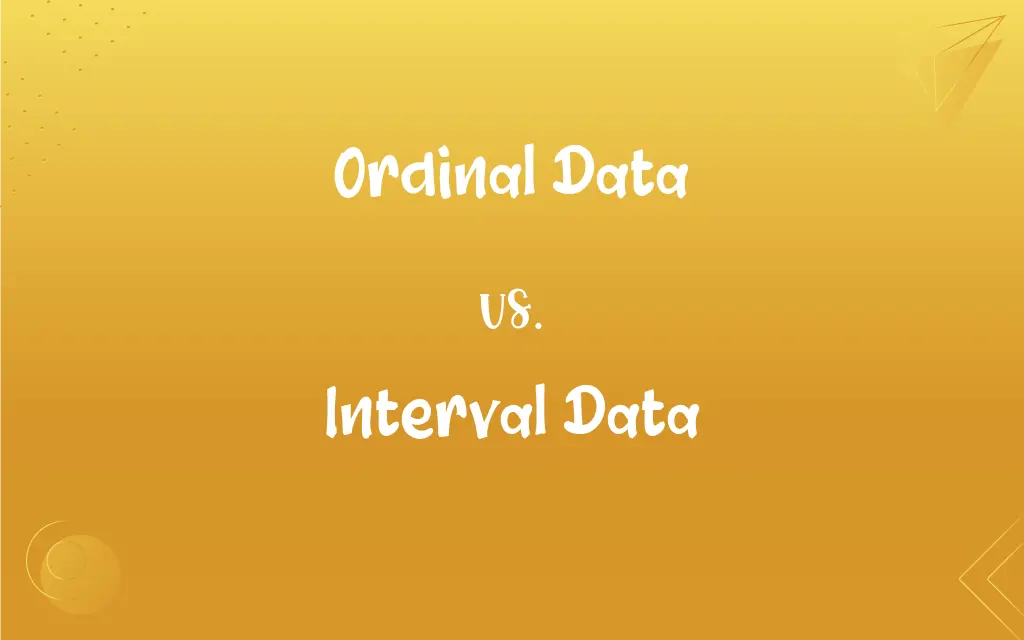# Ordinal Data vs. Interval Data: What's the Difference?Edited by Huma Saeed || By Sawaira Riaz || Updated on October 30, 2023
Ordinal data represents rank order, while interval data has consistent, measurable differences between values without a true zero.## Key Differences

Ordinal data refers to data types where items can be ranked or put in order. In contrast, interval data is quantitative data that has equal and consistent intervals but lacks a genuine starting point or true zero.
When considering ordinal data, one can understand the relative position or ranking of items, but not the magnitude of difference between them. Interval data, on the other hand, allows for the measurement of the exact difference between data points, but it doesn't convey a true sense of "absence" since it lacks a true zero.
For instance, ordinal data might represent how students rank in a class: first, second, third, and so forth. But it won't tell you the exact difference in scores between the first and second student. Conversely, interval data, such as temperature measured in Celsius or Fahrenheit, can tell the exact difference between two values, but 0°C or 0°F doesn't signify the absence of temperature.
To delve deeper, ordinal data might use scales like "agree, neutral, disagree" which provide a clear order but no consistent difference between each response. Interval data like IQ scores or SAT scores provide definite intervals, letting one discern not just order but the exact distance between scores.
It's critical to recognize that while ordinal data gives a sense of hierarchy, it doesn't give a sense of the interval between categories. In contrast, interval data provides a clear picture of the spaces between each data point, yet can't represent an absolute absence of the attribute being measured.

## Comparison Chart

Categorical
Quantitative

### Representation

Rank order
Consistent, measurable differences

### Measurement

Order without exact difference
Precise differences without true zero

### Example

Likert scales: agree, neutral, disagree
Temperature in Celsius or Fahrenheit

Absent
Absent

## Ordinal Data and Interval Data Definitions

#### Ordinal Data

It's categorical data with a clear hierarchy.
Survey responses like low, medium, high represent ordinal data.

#### Interval Data

It allows for arithmetic operations.
With interval data, one can calculate the average of two temperatures.

#### Ordinal Data

Ordinal data allows for relative comparisons.
In a race, ordinal data can tell you who finished first, second, or third, but not the time gaps.

#### Interval Data

The space between data points is uniform.
The difference between 10°C and 20°C in interval data is the same as between 20°C and 30°C.

#### Ordinal Data

It lacks precise numerical differences.
While you know gold is better than silver in a contest using ordinal data, you don't know by how much.

#### Interval Data

Interval data has consistent, measurable differences.
Temperatures on the Fahrenheit scale represent interval data.

#### Ordinal Data

Ordinal data represents a rank order.
Students' class ranks are a form of ordinal data.

#### Interval Data

The data points are quantitative.
IQ scores, measured at regular intervals, are interval data.

#### Ordinal Data

The intervals between data points are not uniform.
The difference between disagree and neutral in ordinal data might not be the same as between neutral and agree.

#### Interval Data

It lacks a true starting point or zero.
In interval data, 0°C doesn't mean the absence of temperature.

## FAQs

#### How does interval data differ?

Interval data has consistent, measurable differences between values but lacks a true zero.

#### Why doesn't interval data have a true zero?

Because 0 in interval data doesn't indicate the absence of the attribute being measured.

#### Can you measure precise differences in ordinal data?

No, ordinal data only conveys rank order, not exact differences.

#### Is ordinal data quantitative or categorical?

Ordinal data is categorical.

#### How is ordinal data typically represented?

Through scales like "low, medium, high" or "agree, neutral, disagree."

#### Give an example of interval data.

Temperature measured in Celsius or Fahrenheit.

#### Is the difference between "strongly agree" and "agree" uniform in ordinal data?

No, ordinal data doesn't guarantee uniform intervals.

#### Can interval data be ranked?

Yes, but it also offers exact differences between values.

#### What is ordinal data?

Ordinal data represents data with a rank order or hierarchy.

#### Why is understanding the difference important?

Different data types require different statistical analyses.

#### What's an example of a dataset with both ordinal and interval data?

A survey with questions about income brackets (ordinal) and age (interval).

#### Can you convert ordinal data to interval data?

Not directly, as ordinal data lacks consistent intervals.

#### Can you calculate the median for both data types?

Yes, both ordinal and interval data can have medians.

#### Can arithmetic operations be performed on interval data?

Yes, operations like averaging can be done with interval data.

#### How is ordinal data visualized?

Commonly through bar charts or pie charts.

#### Is age ordinal or interval data?

Age is typically considered interval data.

#### Why is there no true zero in interval data?

A true zero would mean the complete absence of the attribute, which isn't represented in interval scales.

#### Can ordinal data be numeric?

Yes, like "1st, 2nd, 3rd," but the numbers represent ranks, not measurable quantities.

#### Why can't you say 0°C means no temperature?

Because 0°C is just a point on the scale and doesn't signify the absence of heat.

#### Is a Likert scale ordinal or interval data?

Typically, a Likert scale is treated as ordinal data.